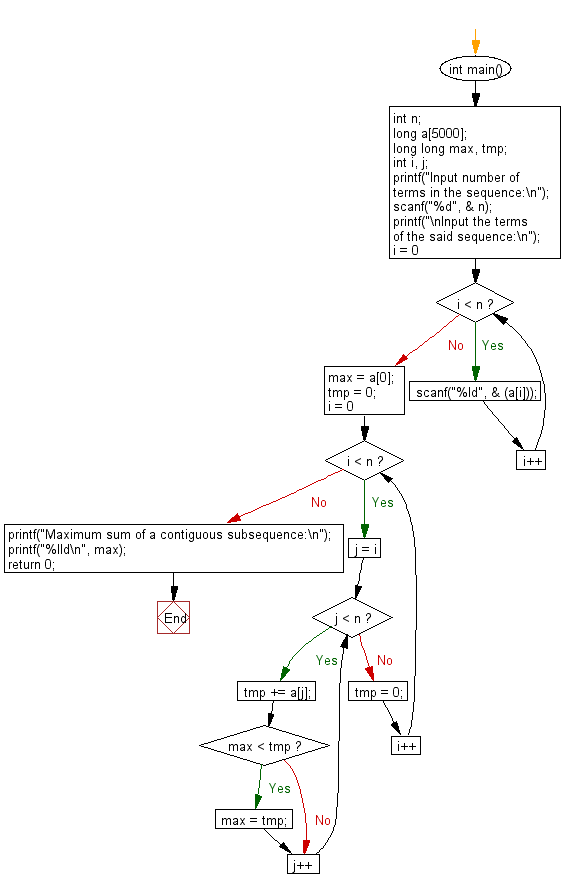﻿ C : The maximum sum of a contiguous subsequence

# C Exercises: Maximum sum of a contiguous subsequence from a given sequence of numbers

## C Basic Declarations and Expressions: Exercise-139 with Solution

Write a C program to find the maximum sum of a contiguous subsequence from a given sequence of numbers a1, a2, a3, ... an ( n = number of terms in the sequence).

You can assume that 1 <= n <= 500 and -10000 <= ai <= 10000.
A contiguous subsequence of a list S is a subsequence made up of consecutive elements of S.
For instance, if S is
5, 15, −30, 10, −5, 40, 10,
then 15, −30, 10 is a contiguous subsequence but 5, 15, 40 is not.
Give a linear-time algorithm for the following task:
• Input: A list of numbers a1, a2, . . . , an
• Output: The contiguous subsequence of maximum sum. (Note that a subsequence of length zero has sum zero.)
For the preceding example, the answer would be 10, −5, 40, 10, with a sum of 55
Ref: https://bit.ly/2IGGI3f

Sample Solution:

C Code:

``````#include <stdio.h>

int main() {
int n;
long a;
long long max, tmp;
int i, j;

// Getting the number of terms in the sequence from the user
printf("Input number of terms in the sequence:\n");
scanf("%d", &n);

// Getting the terms of the sequence from the user
printf("\nInput the terms of the said sequence:\n");
for (i = 0; i < n; i++)
scanf("%ld", &(a[i]));

max = a;
tmp = 0;

// Finding the maximum sum of a contiguous subsequence
for (i = 0; i < n; i++) {
for (j = i; j < n; j++) {
tmp += a[j];
if (max < tmp)
max = tmp;
}
tmp = 0;
}

// Printing the maximum sum of a contiguous subsequence
printf("Maximum sum of a contiguous subsequence:\n");
printf("%lld\n", max);

return 0; // End of the program
}
``````

Sample Output:

```Input number of terms in the sequence:
5

Input the terms of the said sequence:
3
2
6
-7
8
Maximum sum of a contiguous subsequence:
12
```

Flowchart:C programming Code Editor:

What is the difficulty level of this exercise?

Test your Programming skills with w3resource's quiz.

﻿